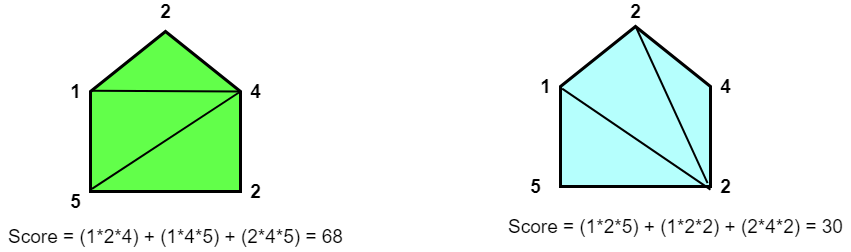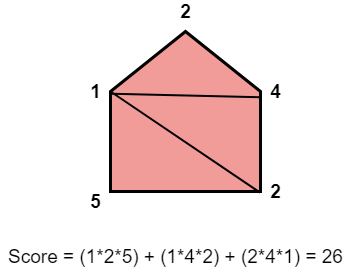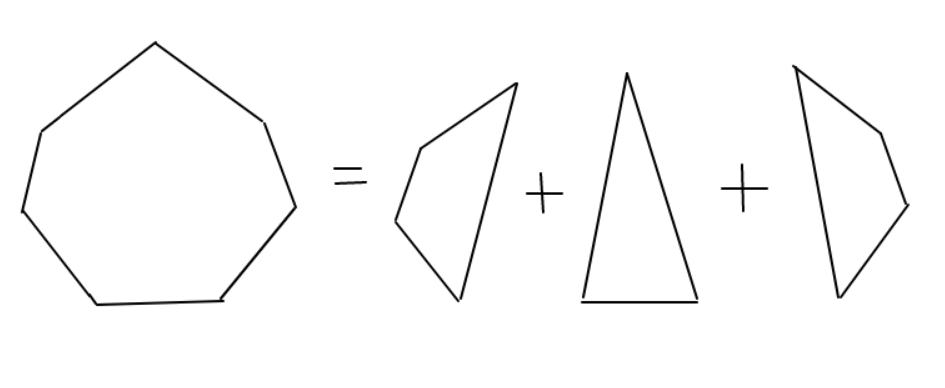# Minimum Score Triangulation of Polygon

## Introduction

In this article, we will solve the problem of finding the minimum score triangulation of polygon by three methods: recursion, memoization with recursion, and finally, we will discuss an iterative DP approach.

Let’s get started with the problem statement.

## Problem Statement

You are given an N sided polygon. Every vertex of the polygon is assigned a value. The vertices are given in the form of an array of integers in the clockwise direction.

You need to divide the polygon into N - 2 triangles. Each triangle has a triangle value. The triangle value is calculated by finding the product of its vertices.

Now, you need to find the minimum total triangle score. The total triangle score is the sum of the triangle scores of all the possible triangles.

Note:

A polygon can be divided into triangles in more than one way. You need to print the minimum sum of triangle values of all the triangles created.

Please try to solve this problem on your own before moving on to further discussion here

Example -

N = 5

vertex[] = {2, 4, 2, 5, 1}

Some of the ways of triangulating are shown below:But we can reduce the score further. The most optimal triangulation to obtain a minimum score is:One possible way to find the minimum score is to try all possible triangulations of the polygon and calculate the scores for each. Then, choose the minimum score among them.

To get all possible triangulations of a given polygon, we will follow the steps below:

• For a triangle, fix an edge of the polygon as one side of the triangle. Now, to get the third vertex, run a loop from i+1 to j-1.

• Calculate the score of the triangle [i,j,k] by multiplying vertex[i], vertex[j] and vertex[k].

• After choosing this triangle, there are two parts of the polygon left to be triangulated. One polygon is on the left side of the triangle and the other on the right, as illustrated below:• Since we got two sub-polygons, then the total score of triangulation of the main polygon will be-
minScore(P) = minScore(P1) + minScore(P2) + vertex[i]*vertex[j]*vertex[k]

• To get the minimum score for polygon P, the scores of polygon P1 and P2 should also be minimised.

• Thus, we got two subproblems to be solved, which implies we can use recursion.

Let’s see the implementation of the recursive approach in the next section.

## C++ Implementation(Recursive)

Output

### Time Complexity

O(n ^ (n - 3)), where n is the number of sides in the polygon.

Since we are traversing through the complete array for (n-3) times (until we reach an array having only 3 sides), the overall time complexity will be O(n ^ (n - 3)).

### Space Complexity

O(1)since we have not used any extra space.

## Recursion with Memoization

In the recursive approach, we are solving many subproblems repetitively. So, to reduce the time complexity, we can store the results of every subproblem, and when its value is needed later, we can simply use its stored value rather than calculate it again. This is called memoization.

The approach remains the same except for the additional 2D DP array to store the results. Let’s see its implementation.

### C++ Implementation

Output

#### Time Complexity

O(n^3), There are (n^2) DP states from dp to dp[n-1][n-1]. It takes linear time to calculate each dp[i][j] as we have to run a loop from k=i+1 to k=j-1.

So, total time complexity becomes -

= Number of dp states * (Time complexity to calculate the result for each state)

= (n^2) * O(n)

= O(n^3).

#### Space Complexity

O(n^2), Since we use a 2D vector to store our previous results, the overall space complexity is O(n^2).

## Dynamic Programming Approach

In this method, we will calculate the results to all the smaller subproblems iteratively and then use these to get the values of larger subproblems which is essentially a bottom-up approach.

The implementation of this approach is quite simple and easy to understand.

### C++ Implementation

Output

#### Time Complexity

O(n^3), as there are three nested loops.

#### Space Complexity

O(n^2), as we are using a 2D DP array of size n*n.

1. What is recursion?
Recursion is a method of solving a problem in terms of similar but smaller subproblems.

2. What is dynamic programming?
Dynamic Programming or DP is just an optimization technique. It is a method for solving problems by breaking them down into a collection of simpler subproblems, solving each of those subproblems just once, and storing their solutions.
Do check out Roadmap For Beginners To Master Dynamic Programming

## Key Takeaways

In this article, we learned how to solve the problem of finding the minimum score triangulation of polygon by three methods: recursion, memoization with recursion, and finally, the iterative DP approach. At first glance, it’s difficult to come up with a DP solution directly, but you will definitely be able to solve these types of problems with more practice.

Problems based on a similar concept that you must give a try -

Are you planning to ace the interviews of reputed product-based companies like Amazon, Google, Microsoft, and more? Attempt our Online Mock Test Series on CodeStudio now!